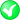#Boost C++ Libraries

...one of the most highly regarded and expertly designed C++ library projects in the world.

#### append

Appends one or more points to a linestring, ring, polygon, multi-geometry.

###### Synopsis

```template<typename Geometry, typename RangeOrPoint>
void append(Geometry & geometry, RangeOrPoint const & range_or_point, int ring_index = -1,
int multi_index = 0)```

###### Parameters

Type

Concept

Name

Description

Geometry &

Any type fulfilling a Geometry Concept

geometry

A model of the specified concept

RangeOrPoint const &

Either a range or a point, fullfilling Boost.Range concept or Boost.Geometry Point Concept

range_or_point

The point or range to add

int

ring_index

The index of the ring in case of a polygon: exterior ring (-1, the default) or interior ring index

int

multi_index

The index of the geometry to which the points are appended

Either

`#include <boost/geometry.hpp>`

Or

`#include <boost/geometry/algorithms/append.hpp>`

###### Conformance

The function append is not defined by OGC.

###### Supported geometries

Point

Range

PointSegmentBoxLinestringRingPolygonMultiPointMultiLinestringMultiPolygon###### Behavior

Case

Behavior

Point, Box, Segment

Compiles, but no action

Linestring

Appends point or range to the end of the linestring

Ring

Appends point or range to the end of the ring (without explicitly closing it)

Polygon

Appends point or range to the end of the polygon (without explicitly closing it), either the exterior ring (the default) or specify a zero-based index for one of the interior rings. In the last case, the interior rings are not resized automatically, so ensure that the zero-based index is smaller than the number of interior rings

Multi Linestring

Appends point or range to the end of the linestring with the given multi index. The multi-linestring is not resized automatically, so ensure that the multi index is smaller than then number of linestring in the multi-linestring.

Multi Polygon

Appends point or range to the end of the polygon (without explicitly closing it) with the given multi-index. The point or range is appended at the end of the exterior ring (the default) or specify a zero-based ring index for the interior rings. The multi-polygon is not resized automatically, so ensure that the multi index is smaller than then number of polygon in the multi-polygon. The same applies for the interior rings of the polygon: the interior rings are not resized automatically, so ensure that the zero-based ring index is smaller than the number of interior rings of the polygon.

Linear

###### Example

Shows usage of Boost.Geometry's append to append a point or a range to a polygon

```#include <iostream>

#include <boost/geometry.hpp>
#include <boost/geometry/geometries/polygon.hpp>

#include <boost/assign.hpp>BOOST_GEOMETRY_REGISTER_BOOST_TUPLE_CS(cs::cartesian)

int main()
{
using boost::assign::tuple_list_of;
using boost::make_tuple;
using boost::geometry::append;

typedef boost::geometry::model::polygon<boost::tuple<int, int> > polygon;

polygon poly;

// Append a range
append(poly, tuple_list_of(0, 0)(0, 10)(11, 11)(10, 0));// Append a point (in this case the closing point)
append(poly, make_tuple(0, 0));

// Create an interior ring (append does not do this automatically)
boost::geometry::interior_rings(poly).resize(1);

// Append a range to the interior ring
append(poly, tuple_list_of(2, 2)(2, 5)(6, 6)(5, 2), 0);// Append a point to the first interior ring
append(poly, make_tuple(2, 2), 0);

std::cout << boost::geometry::dsv(poly) << std::endl;

return 0;
}
```

 At the end to avoid conflicts with Boost.QVM tuple_list_of delivers a range and can therefore be used in boost::geometry::append The last parameter ring_index 0 denotes the first interior ring

Output:

```(((0, 0), (0, 10), (11, 11), (10, 0), (0, 0)), ((2, 2), (2, 5), (6, 6), (5, 2), (2, 2)))
```